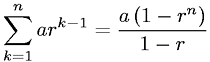Equations > Calculus > Sequences and Series > Finite Geometric Series

### Finite Geometric SeriesLatex Code:

MathML Code:

 $\sum _{k=1}^{n}{\mathrm{ar}}^{k-1}=\fraca\left(1-{r}^{n}\right)1-r$

MathType 5.0: